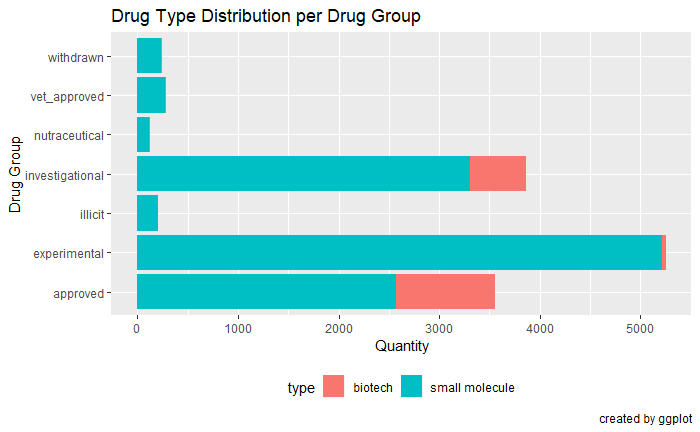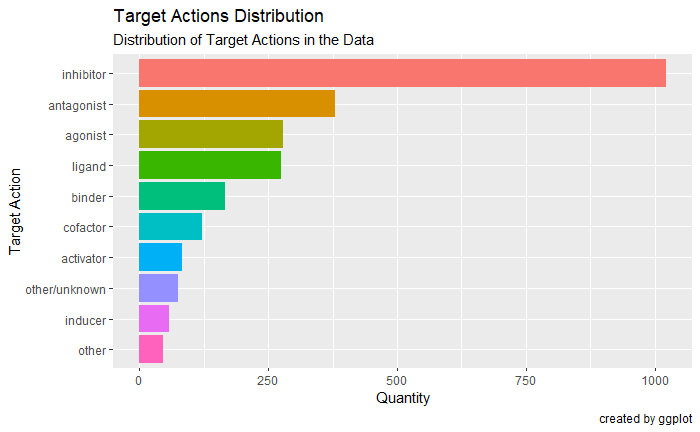# DrugBank Database XML Parser

## Introduction

The main purpose of the dbparser package is to parse the DrugBank database which is downloadable in XML format from this link. The parsed data can then be explored and analyzed as desired by the user. In this tutorial, we will see how to use dbparser along with dplyr and ggplot2 along with other libraries to do simple drug analysis

Before starting the code we are assuming the following:

• user saved the downloaded database in working directory as C:\.

Now we can loads the drugs info, drug groups info and drug targets actions info.

## load dbparser package
library(dbparser)
library(dplyr)
library(ggplot2)
library(XML)

## parse data from XML and save it to memory
dvobj <- parseDrugBank(db_path            = "C:\drugbank.xml",
drug_options       = drug_node_options(),
parse_salts        = TRUE,
parse_products     = TRUE,
references_options = references_node_options(),
cett_options       = cett_nodes_options())

drugs <- dvobj$drugs$general_information

drug_groups <- dvobj$drugs$groups

## load drug targets actions data
drug_targets_actions <- dvobj$cett$targets\$actions

## Exploring the data

Following is an example involving a quick look at a few aspects of the parsed data. First we look at the proportions of biotech and small-molecule drugs in the data.

## view proportions of the different drug types (biotech vs. small molecule)
drugs %>%
select(type) %>%
ggplot(aes(x = type, fill = type)) +
geom_bar() +
guides(fill = FALSE)     ## removes legend for the bar colors

Below, we view the different drug_groups in the data and how prevalent they are.

## view proportions of the different drug types for each drug group
drugs %>%
full_join(drug_groups, by = c('primary_key' = 'drugbank_id')) %>%
select(type, group) %>%
ggplot(aes(x = group, fill = type)) +
geom_bar() +
theme(legend.position = 'bottom') +
labs(x = 'Drug Group',
y = 'Quantity',
title = "Drug Type Distribution per Drug Group",
caption = "created by ggplot") +
coord_flip()

Finally, we look at the drug_targets_actions to observe their proportions as well.## get counts of the different target actions in the data
targetActionCounts <-
drug_targets_actions %>%
group_by(action) %>%
summarise(count = n()) %>%
arrange(desc(count))

## get bar chart of the 10 most occurring target actions in the data
p <-
ggplot(targetActionCounts[1:10,],
aes(x = reorder(action,count), y = count, fill = letters[1:10])) +
geom_bar(stat = 'identity') +
labs(fill = 'action',
x = 'Target Action',
y = 'Quantity',
title = 'Target Actions Distribution',
subtitle = 'Distribution of Target Actions in the Data',
caption = 'created by ggplot') +
guides(fill = FALSE) +    ## removes legend for the bar colors
coord_flip()              ## switches the X and Y axes

## display plot
p